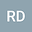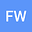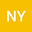Existence of positive solutions of second-order delayed differential system on infinite interval
•••• +1
• Ran Ding,
• Fanglei Wang,
• Nannan Yang,
• Yuanfang RU
Ran Ding
Hohai University

Corresponding Author:randing98@163.com

Author ProfileFanglei Wang
College of Science, Hohai University
Author ProfileNannan Yang
Hohai University
Author ProfileYuanfang RU
China Pharmaceutical University
Author Profile## Abstract

The present paper is focused on the analysis on the existence of positive solutions of a second order differential system with two delays

\begin{equation} \left\{\begin{array}{lcr}x_{1}^{\prime\prime}(t)-a_{1}(t)x_{1}(t)+m_{1}(t)f_{1}(t,x(t),x_{\tau}(t))=0,\ \ t>0,\\ x_{2}^{\prime\prime}(t)-a_{2}(t)x_{2}(t)+m_{2}(t)f_{2}(t,x(t),x_{\tau}(t))=0,\ \ t>0,\\ x_{1}(t)=0,\ \ -\tau_{1}\ \leq t\leq 0,\;\text{and}\;\lim_{t\rightarrow\infty}x_{1}(t)=0,\\ x_{2}(t)=0,\ \ -\tau_{2}\ \leq t\leq 0,\;\text{and}\;\lim_{t\rightarrow\infty}x_{2}(t)=0\end{array}\right.\nonumber \\ \end{equation}

by using two well-known fixed point theorems.

23 Feb 2020Submitted to Mathematical Methods in the Applied Sciences
28 Feb 2020Submission Checks Completed
28 Feb 2020Assigned to Editor
10 Jul 2020Reviewer(s) Assigned
12 Jan 2021Review(s) Completed, Editorial Evaluation Pending
12 Jan 2021Editorial Decision: Revise Minor